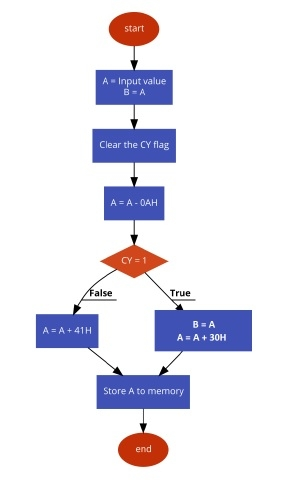# Program to convert HEX to ASCII in 8085 Microprocessor

Here we will see one 8085 Microprocessor program. That program will convert HEX to ASCII values.

Problem Statement

Write an 8085 Assembly language program to convert Hexadecimal characters to ASCII values.

Discussion

We know that the ASCII of number 00H is 30H (48D), and ASCII of 09H is 39H (57D). So all other numbers are in the range 30H to 39H. The ASCII value of 0AH is 41H (65D) and ASCII of 0FH is 46H (70D), so all other alphabets (B, C, D, E, F) are in the range 41H to 46H.

Here we are providing hexadecimal digit at memory location 8000H, The ASCII equivalent is storing at location 8001H.

The logic behind HEX to ASCII conversion is very simple. We are just checking whether the number is in range 0 – 9 or not. When the number is in that range, then the hexadecimal digit is numeric, and we are just simply adding 30H with it to get the ASCII value. When the number is not in range 0 – 9, then the number is range A – F, so for that case, we are converting the number to 41H onwards.

In the program at first we are clearing the carry flag. Then subtracting 0AH from the given number. If the value is numeric, then after subtraction the result will be negative, so the carry flag will be set. Now by checking the carry status we can just add 30H with the value to get ASCII value.

In other hand when the result of subtraction is positive or 0, then we are adding 41H with the result of the subtraction.

first input

Data

8000
0A

second input

Data

8000
05

third input

Data

8000
0F

## Flow Diagram## Program

HEX Codes
Labels
Mnemonics
F000
21, 00, 80

LXI H, 8000H
F003
7E

MOV A,M
Load Acc with the data from memory
F004
47

MOV B,A
Copy the number into B
F005
37

STC
Set Carry Flag
F006
3F

CMC
Complement Carry Flag
F007
D6, 0A

SUI 0AH
Subtract 0AH from A
F009
DA, 11, F0

JC NUM
When carry is present, A is numeric
F00C
C6, 41

F00E
C3, 14, F0

JMP STORE
F011
78
NUM
MOV A, B
Get back B to A
F012
C6

Add 30H with A to get ASCII
F014
23
STORE
INX H
Point to next location to store address
F015
77

MOV M,A
Store A to memory location pointed by HL pair
F016
76

HLT
Terminate the program

first output

Data

8001
41

second output

Data

8001
35

third output